# How to Find the Period of Cosine Functions

Lesson Transcript
Instructor
Laura Pennington

Laura received her Master's degree in Pure Mathematics from Michigan State University, and her Bachelor's degree in Mathematics from Grand Valley State University. She has 20 years of experience teaching collegiate mathematics at various institutions.

Expert Contributor
Kathryn Boddie

Kathryn has taught high school or university mathematics for over 10 years. She has a Ph.D. in Applied Mathematics from the University of Wisconsin-Milwaukee, an M.S. in Mathematics from Florida State University, and a B.S. in Mathematics from the University of Wisconsin-Madison.

This lesson shows how to find the period of cosine functions. We then take it a step further and look at the amplitude, phase shift, and vertical shift of a cosine function and how to find all of these characteristics of cosine functions. Updated: 12/04/2019

## Steps to Solve

The cosine function is a trigonometric function that's called periodic. In mathematics, a periodic function is a function that repeats itself over and over again forever in both directions. Take a look at the basic cosine function f(x) = cos(x).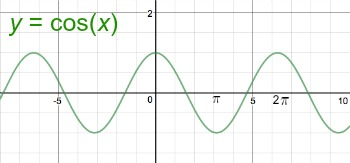If we look at the cosine function from x = 0 to x = 2π, we have an interval of the graph that's repeated over and over again in both directions, so we can see why the cosine function is a periodic function.

This interval from x = 0 to x = 2π of the graph of f(x) = cos(x) is called the period of the function. The period of a periodic function is the interval of x-values on which the cycle of the graph that's repeated in both directions lies. Therefore, in the case of the basic cosine function, f(x) = cos(x), the period is 2π.

Wouldn't it be easy if that were the end of the story? Easy, yes, but much less interesting! You see, the cosine function takes on many forms, expressed as:

f(x) = Acos(Bx + C) + D

where A, B, C, and D are numbers, and the periods of these cosine functions differ. Thankfully, finding the period of these functions is still quite simple. It all depends on the value of B in the function f(x) = Acos(Bx + C) + D, where B is the coefficient of x. This is because the period of this function is 2π / |B|.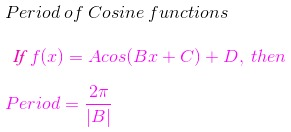To find the period of f(x) = Acos(Bx + C) + D, we follow these steps:

1. Identify the coefficient of x as B.
2. Plug B into 2π / |B|. This is the period of the function.

Let's now consider an example. Suppose we want to find the period of the function g(x) = 3cos(8x + 1). The first thing we would do is find the coefficient of x, which is 8, and take B = 8. Next, we plug B = 8 into the period formula. We end up getting:

Period = 2π / |B| = 2π / |8| = 2π / 8 = π / 4

We see that the period of the function g(x) = 3cos(8x + 1) is π / 4.

An error occurred trying to load this video.

Try refreshing the page, or contact customer support.

Coming up next: How to Find the Period of Sine Functions

### You're on a roll. Keep up the good work!

Replay
Your next lesson will play in 10 seconds
• 0:04 Steps to Solve
• 2:35 Application
• 4:32 Lesson Summary
Save Save

Want to watch this again later?

Timeline
Autoplay
Autoplay
Speed Speed

## Application

Because of the nature of the cosine function, it can be used to model anything in the real world having simple harmonic motion, where simple harmonic motion is described as moving back and forth in a constant fashion with no friction involved. Some examples of this could be a pendulum on a clock, springs, or alternating currents.

For example, suppose you hang a spring from your ceiling that you are considering using to hang a potted plant from. Without the plant on it, when you compress the spring 2 inches and let it go, its motion can be modeled by the following cosine function:

• y = 2 cos ((3 π / 2)x)

where y is the displacement of the end of the spring, and x is the time in seconds.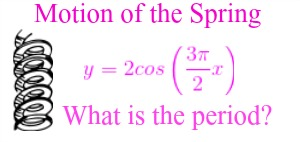To unlock this lesson you must be a Study.com Member.

## Shifting or stretching Cosine Functions

In the video lesson we learned that for a cosine function {eq}f(x)=A\cos(Bx+C)+D {/eq}, the period of the cosine function is {eq}\dfrac{2\pi}{|B|} {/eq}. But what do the other numbers ({eq}A, C, D {/eq}) do to the graph of the cosine function?

## Example

Consider the functions: {eq}f(x)=\cos(2x)\\ g(x)=3\cos(2x)\\ h(x)=\cos(2x+\pi)\\ j(x)=\cos(2x)-1 {/eq}

1) What is the period of these functions?

2) Graph all four functions on the same coordinate plane. This can be done using a graphing calculator or online graphing tool, or by hand by picking values for {eq}x {/eq} and plotting points.

3) How did the graphs of {eq}g(x), h(x), j(x) {/eq} differ from the graph of {eq}f(x) {/eq}?

## Solutions

1) All of the functions are of the form, {eq}y=A\cos(Bx+C)+D {/eq}, and all of the functions have the same {eq}B {/eq} value of {eq}2 {/eq}. The period of a cosine function is given by {eq}\dfrac{2\pi}{|B|} {/eq}, so all four functions have a period of {eq}\dfrac{2\pi}{2}=\pi {/eq}

2)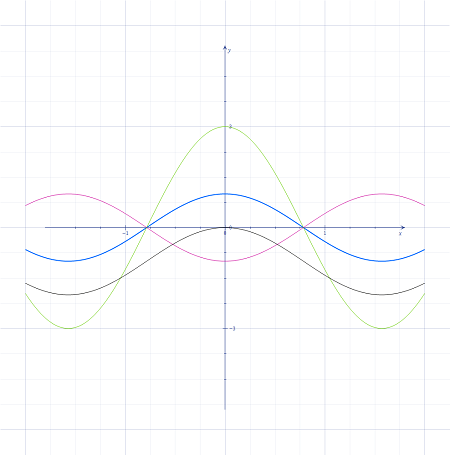3) All of the graphs are similar looking - but the graph of {eq}g(x)=3\cos(2x) {/eq} is taller - it appears that multiplying by {eq}3 {/eq} stretched the graph vertically. The graph of {eq}h(x)=\cos(2x+\pi) {/eq} looks just like the graph of {eq}f(x)=\cos(2x) {/eq}, but shifted to the side {eq}\pi {/eq} units. The graph of {eq}j(x)=\cos(2x)-1 {/eq} looks like the graph of {eq}f(x)=\cos(2x) {/eq}, shifted down one unit.

## Explanation

For a function of the form, {eq}f(x)=A\cos(Bx+C)+D {/eq}, the number, {eq}A {/eq}, vertically stretches the graph of {eq}\cos(Bx) {/eq} by a factor of {eq}A {/eq}. The number, {eq}C {/eq}, shifts the graph of {eq}\cos(Bx) {/eq} horizontally {eq}C {/eq} units to the left, if {eq}C>0 {/eq} and {eq}C {/eq} units to the right if {eq}C<0 {/eq}. The number, {eq}D {/eq}, shifts the graph of {eq}\cos(Bx) {/eq} vertically {eq}D {/eq} units up if {eq}D>0 {/eq} and {eq}D {/eq} units down if {eq}D<0 {/eq}

## Questions to Check Understanding

1) What will the graph of {eq}g(x)=4\cos(3x-4)+1 {/eq} look like compared to the graph of {eq}f(x) = \cos(3x) {/eq}?

2) What are the periods of these functions?

## Solutions

1) The graph of {eq}g(x) {/eq} will be shifted right {eq}4 {/eq} units, vertically stretched by a factor of {eq}4 {/eq}, and shifted up {eq}1 {/eq} unit.

2) Both functions have a period of {eq}\dfrac{2\pi}{3} {/eq}

3)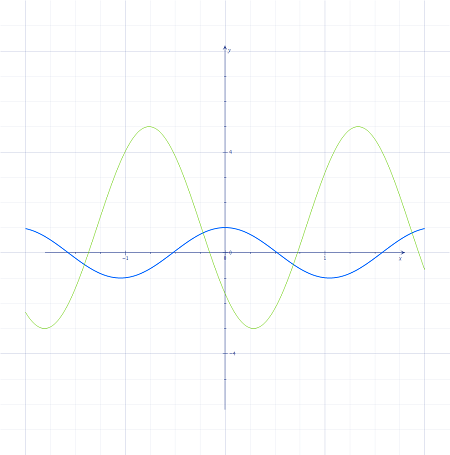### Register to view this lesson

Are you a student or a teacher?